Definitions of Square Dance Calls and Concepts

Index -->  Plus  |  A1  |  A2  |  C1  |  C2  |  C3A  |  C3B  |  C4  |  NOL  |
Definitions (Text Only) -->  Plus  |  A1  |  A2  |  C1  |  C2  |  C3A  |  C3B  |  C4  |  NOL  |
 Find call:

 \$B8@8l(B3/4 Line \$B\$^\$?\$OB>\$NE,@Z\$J(B formation \$B\$+\$i(B.

Outsides \$B\$O(B Cast Back \$B\$r\$7(B, Centers \$B\$O(B Once Removed Fold (Any Shoulder Turn & Deal \$B\$N\$h\$&\$KF0\$-\$^\$9(B) \$B\$r\$7(B; \$BA40w\$G(B Slide Thru \$B\$r\$7\$^\$9(B.

3/4 Line \$B\$+\$i\$O0lHL2=\$5\$l\$?(B Column \$B\$G=*\$o\$j\$^\$9(B. \$B\$3\$l\$O(B 2 \$B%Q!<%H\$N%3!<%k\$G\$9(B.

\$B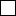= \$BCK@-(B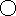= \$B=w@-\$G\$9(B.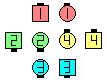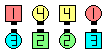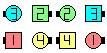Interlocked Ramble\$B\$NA0(B Outsides Cast Back as Centers Once Removed Fold(1/2)\$B\$N8e(B Slide Thru\$B\$N8e(B (\$B=*\$o\$j(B)

\$BCm
• Outsides \$B\$OIaDL\$N(B Ramble [C1] \$B\$r\$7\$^\$9(B.
• \$B\$"\$k;qNA\$G\$O(B Ramble \$B\$N(B Outsides \$B\$NF0\$-\$r(B Cast Back (\$B\$^\$?\$O(B Peel Off) \$B\$G\$J\$/(B, Divide (1/4 Out & Ends Bend) \$B\$HDj5A\$7\$F\$\$\$^\$9(B. \$B\$3\$N\$3\$H\$K\$h\$j(B, Interlocked Ramble \$B\$G(B Outside \$B\$,Cf\$r8~\$\$\$F\$\$\$k\$H\$3\$m\$+\$i\$N%3!<%k\$K=P2q\$&\$3\$H\$,\$"\$k\$+\$bCN\$l\$^\$;\$s(B.
• Ramble [C1] (Norm Poisson 1973): 3/4 Tag \$B\$^\$?\$OB>\$NE,@Z\$J(B formation \$B\$+\$i(B. Outsides \$B\$O(B Cast Back \$B\$r\$7(B, Centers \$B\$O(B Fold \$B\$r\$7\$F\$*8_\$\$\$K8~\$+\$\$9g\$\$(B (Single Wheel \$B\$N\$h\$&\$KF0\$-\$^\$9(B); \$BA40w\$G(B Slide Thru \$B\$r\$7\$^\$9(B. 3/4 Tag \$B\$+\$i\$O0lHL2=\$5\$l\$?(B Column \$B\$G=*\$o\$j\$^\$9(B.Choreography for Interlocked RambleComments? Questions? Suggestions?

https://www.ceder.net/def/interlockedramble.php?level=master&language=japan
31-May-2020 17:44:16